Theory and Modern Applications

# Numerical stability and oscillation of the Runge-Kutta methods for equation ${x}^{\prime }\left(t\right)=ax\left(t\right)+{a}_{0}x\left(M\left[\frac{t+N}{M}\right]\right)$

## Abstract

This paper deals with the numerical properties of Runge-Kutta methods for the alternately of retarded and advanced equation ${x}^{\prime }\left(t\right)=ax\left(t\right)+{a}_{0}x\left(M\left[\frac{t+N}{M}\right]\right)$. Necessary and sufficient conditions for the stability and oscillation of the numerical solution are given. The conditions that the Runge-Kutta methods preserve the stability and oscillations of the analytic solutions are obtained. Some numerical experiments are illustrated.

## 1 Introduction

This paper deals with the numerical solutions of the alternately of retarded and advanced equation with piecewise continuous arguments (EPCA)

${x}^{\prime }\left(t\right)=f\left(x\left(t\right),x\left(M\left[\frac{t+N}{M}\right]\right)\right),$
(1.1)

where $\left[\cdot \right]$ is the greatest integer function, and M and N are positive integers such that $N. Differential equations of this form have simulated considerable interest and have been studied by Wiener and Aftabizadeh . Cooke and Wiener  studied the special cases of this form with $M=1$, $N=\frac{1}{2}$ and $M=2$, $N=1$. In these equations the argument derivation $T\left(t\right)=t-M\left[\frac{t+N}{M}\right]$ are piecewise linear periodic function with periodic M. Also, $T\left(t\right)$ is negative for $Mn-N\le t and positive for $Mn (n is integer). Therefore, Eq. (1.1) is of advanced type on $\left[Mn-N,Mn\right)$ and of retarded type on $\left(Mn,M\left(n+1\right)-N\right)$.

EPCA describe hybrid dynamics systems, combine properties of both differential and difference equations, and have applications in certain biomedical models in the work of Busenberg and Cooke . For these equations of mixed type, the change of sign in the argument derivation leads not only to interesting periodic properties but also to complications in the asymptotic and oscillatory behavior of solutions. Oscillatory, stability and periodic properties of the linear EPCA of the form with alternately of retarded and advanced have been investigated in .

There exist some papers concerning the stability of the numerical solutions of delay differential equations with piecewise continuous arguments, such as [5, 8, 12]. Also, there have been results concerning oscillations of delay differential equations and delay difference equations, even including delay differential equations with piecewise continuous arguments . But there is no paper concerning with the stability and oscillation of the numerical solution of these equations.

In this paper, we will investigate the numerical properties, including the stability and oscillation of Runge-Kutta methods for equation ${x}^{\prime }\left(t\right)=ax\left(t\right)+{a}_{0}x\left(M\left[\frac{t+N}{M}\right]\right)$. In Section 2 we give some preliminary results for the analytic solutions of this equation. In Section 3 we consider the adaptation of the Runge-Kutta methods. In Section 4 we give the conditions of the stability and oscillation of the Runge-Kutta methods. In Section 5 we investigate the preservation of the stability and oscillations of the Runge-Kutta methods. In Section 6 some numerical experiments are illustrated.

## 2 Preliminary results

We consider the following equation

$\left\{\begin{array}{c}{x}^{\prime }\left(t\right)=ax\left(t\right)+{a}_{0}x\left(M\left[\frac{t+N}{M}\right]\right),\hfill \\ x\left(0\right)={x}_{0},\hfill \end{array}$
(2.1)

where a, ${a}_{0}$ are constants, M and N are positive integers such that $N ($M\ne 2N$) and $\left[\cdot \right]$ is the greatest integer function.

Definition 2.1

A solution of Eq. (2.1) on $\left[0,\mathrm{\infty }\right)$ is a function $x\left(t\right)$ that satisfies the conditions:

1. 1.

$x\left(t\right)$ is continuous on $\left[0,\mathrm{\infty }\right)$.

2. 2.

The derivative ${x}^{\prime }\left(t\right)$ exists at each point $t\in \left[0,\mathrm{\infty }\right)$ with the possible exception of the points $t=Mn-N$ ($n=1,2,\dots$), where one-sided derivatives exist.

3. 3.

Eq. (2.1) is satisfied on each interval $\left[Mn-N,M\left(n+1\right)-N\right)$ for $n=1,2,\dots$ .

As follows, we use these notations

$\lambda \left(t\right)={e}^{at}+\left({e}^{at}-1\right){a}^{-1}{a}_{0},\phantom{\rule{2em}{0ex}}{\lambda }_{1}=\lambda \left(M-N\right),\phantom{\rule{2em}{0ex}}{\lambda }_{-1}=\lambda \left(-N\right).$

The following theorems give the existence and uniqueness of solutions and then provide necessary and sufficient conditions for the asymptotic stability and the oscillation of all solutions of Eq. (2.1).

Theorem 2.2

Assume that$a,{a}_{0},{x}_{0}\in \mathbb{R}$, then the initial value problem (2.1) has on$\left[0,\mathrm{\infty }\right)$a unique solution$x\left(t\right)$given by

$x\left(t\right)=\lambda \left(T\left(t\right)\right){\left(\frac{{\lambda }_{1}}{{\lambda }_{-1}}\right)}^{\left[\frac{t+N}{M}\right]}{x}_{0},$
(2.2)

if${\lambda }_{-1}\ne 0$, where$T\left(t\right)=t-M\left[\frac{t+N}{M}\right]$.

Theorem 2.3

The solution$x=0$of Eq. (2.1) is asymptotically stable as$t\to +\mathrm{\infty }$if and only if$|\frac{{\lambda }_{1}}{{\lambda }_{-1}}|<1$.

Lemma 2.4

For$M\ne 2N$, the equation

$\phi \left(a\right)\equiv {e}^{Ma}-2{e}^{Na}+1=0$
(2.3)

has a unique nonzero solution with respect to a. This solution is negative if$M>2N$and positive if$M<2N$.

Theorem 2.5

Let${a}^{\ast }$be the nonzero solution of (2.3). The solution$x=0$of Eq. (2.1) is asymptotically stable ($x\left(t\right)\to 0$as$t\to +\mathrm{\infty }$) if any one of the following hypotheses is satisfied:

(${A}_{1}$) $a>{a}^{\ast }$, $\frac{-a\left({e}^{Ma}+1\right)}{\phi \left(a\right)}<{a}_{0}<-a$;

(${A}_{2}$) $a<{a}^{\ast }$, ${a}_{0}>\frac{-a\left({e}^{Ma}+1\right)}{\phi \left(a\right)}$or${a}_{0}<-a$;

(${A}_{3}$) $a={a}^{\ast }$, ${a}_{0}<-a$.

In the following, we will give the definition of the oscillation and the non-oscillation.

Definition 2.6 A nontrivial solution of (2.1) is said to be oscillatory if there exists a sequence $\left\{{t}_{k}\right\}$ such that ${t}_{k}\to \mathrm{\infty }$ as $k\to \mathrm{\infty }$ and $x\left({t}_{k}\right)x\left({t}_{k-1}\right)\le 0$. Otherwise it is called non-oscillatory. We say Eq. (2.1) is oscillatory if all nontrivial solutions of Eq. (2.1) are oscillatory. We say Eq. (2.1) is non-oscillatory if all nontrivial solutions of Eq. (2.1) are non-oscillatory.

Theorem 2.7

A necessary and sufficient condition for all solutions of Eq. (2.1) to be oscillatory is that either of the conditions${a}_{0}<-\frac{a}{{e}^{a\left(M-N\right)}-1}{e}^{a\left(M-N\right)}$, ${a}_{0}>\frac{a}{{e}^{aN}-1}$holds.

## 3 The Runge-Kutta methods

In this section we consider the adaptation of the Runge-Kutta methods $\left(A,b,c\right)$. Let $h=\frac{1}{m}$ be a given stepsize with integer $m\ge 1$ and the gridpoints be defined by ${t}_{n}=nh$ ($n=0,1,2,\dots$).

For the Runge-Kutta methods we always assume that ${b}_{1}+{b}_{2}+\cdots +{b}_{\nu }=1$ and $0\le {c}_{1}\le {c}_{2}\le \cdots \le {c}_{\nu }\le 1$.

The adaptation of Runge-Kutta methods to Eq. (2.1) leads to a numerical process of the following type

$\left\{\begin{array}{c}{x}_{n+1}={x}_{n}+h{\sum }_{i=1}^{\nu }{b}_{i}\left(a{y}_{i}^{n}+{a}_{0}{z}_{i}^{n}\right),\hfill \\ {y}_{i}^{n}={x}_{n}+h{\sum }_{j=1}^{\nu }{a}_{ij}\left(a{y}_{i}^{n}+{a}_{0}{z}_{i}^{n}\right),\hfill \end{array}$
(3.1)

where matrix $A={\left({a}_{ij}\right)}_{\nu ×\nu }$, vectors $b={\left({b}_{1},{b}_{2},\dots ,{b}_{\nu }\right)}^{T}$, $c={\left({c}_{1},{c}_{2},\dots ,{c}_{\nu }\right)}^{T}$ and ${x}_{n}$ is an approximation to $x\left(t\right)$ at ${t}_{n}$, ${y}_{i}^{n}$ and ${z}_{i}^{n}$ are approximation to $x\left({t}_{n}+{c}_{i}h\right)$ and $x\left(M\left[\frac{{t}_{n}+{c}_{i}h+N}{M}\right]\right)$, respectively.

Let $n=Mkm+l$, $l=-Nm,-Nm+1,\dots ,\left(M-N\right)m-1$ for $k\ge 1$; $l=0,1,\dots ,\left(M-N\right)m-1$ for $k=0$. Then ${z}_{i}^{\left(Mkm+l\right)}$ can be defined as ${x}_{Mkm}$ according to Definition 2.1 ($i=1,\dots ,\nu$).

Let ${Y}^{n}=\left({y}_{1}^{n},{y}_{2}^{n},\dots ,{y}_{\nu }^{n}\right)$. Then (3.1) reduces to

$\left\{\begin{array}{c}{x}_{Mkm+l+1}={x}_{Mkm+l}+ha{b}^{T}{Y}^{Mkm+l}+h{a}_{0}{x}_{Mkm},\hfill \\ {Y}^{Mkm+l}={x}_{Mkm+l}e+haA{Y}^{Mkm+l}+h{a}_{0}Ae{x}_{Mkm},\hfill \end{array}$
(3.2)

where $e={\left(1,1,\dots ,1\right)}^{T}$. Hence we have

${x}_{Mkm+l+1}=R\left(x\right){x}_{Mkm+l}+\frac{{a}_{0}}{a}\left(R\left(x\right)-1\right){x}_{Mkm},$
(3.3)

where $x=ha$, $R\left(x\right)=1+x{b}^{T}{\left(I-xA\right)}^{-1}e$ is the stability function of the method.

We can obtain from (3.3)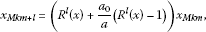(3.4)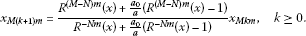(3.5)

## 4 Stability and oscillation of the Runge-Kutta methods

In this section we will discuss stability and oscillation of the Runge-Kutta methods.

### 4.1 Numerical stability

Definition 4.1 The Runge-Kutta method is called asymptotically stable at $\left(a,{a}_{0}\right)$ if there exists a constant C such that Eq. (3.1) defines ${x}_{n}$ ($n\ge 1$) that satisfy ${lim}_{n\to \mathrm{\infty }}{x}_{n}=0$ for all $h=\frac{1}{m}$ ($m>C$) and any given ${x}_{0}$.

For any given Runge-Kutta methods, $R\left(x\right)=\frac{P\left(x\right)}{Q\left(x\right)}$, where $P\left(x\right)$ and $Q\left(x\right)$ are polynomials. $R\left(x\right)$ is a continuous function at the neighborhood of zero, and $R\left(0\right)={R}^{\prime }\left(0\right)=1$, so there exist ${\delta }_{1}$, ${\delta }_{2}$ with ${\delta }_{1}<0<{\delta }_{2}$ such that

$\left\{\begin{array}{cc}1
(4.1)

which implies $0<\frac{R\left(x\right)-1}{x}<\mathrm{\infty }$, ${\delta }_{1}.

Remark 4.2

It is known that $R\left(x\right)$ is an increasing function in $\left[-1,1\right]$, and $1 for $0, $0 for $-1\le x<0$. Hence we can take ${\delta }_{1}=-1$, ${\delta }_{2}=1$ for simplicity.

In the following, we always suppose $h<\frac{1}{|a|}$, i.e., $C=|a|$. It is easy to see from (3.4) and (3.5) that ${x}_{n}\to 0$ as $n\to \mathrm{\infty }$ if and only if ${x}_{Mkm}\to 0$ as $k\to \mathrm{\infty }$. Hence we have the following theorem.

Theorem 4.3 The Runge-Kutta method is asymptotically stable if any one of the following hypotheses is satisfied:

(${N}_{1}$) $\frac{-a\left({R}^{Mm}\left(x\right)+1\right)}{\overline{\phi }\left(x\right)}<{a}_{0}<-a$, $a>\overline{a}$;

(${N}_{2}$) ${a}_{0}>\frac{-a\left({R}^{Mm}\left(x\right)+1\right)}{\overline{\phi }\left(x\right)}$or${a}_{0}<-a$, $a<\overline{a}$;

(${N}_{3}$) ${a}_{0}<-a$, $a=\overline{a}$,

where$x=ha$, $\overline{\phi }\left(x\right)\equiv {R}^{Mm}\left(x\right)-2{R}^{Nm}\left(x\right)+1$, $h\overline{a}$is a nonzero solution of$\overline{\phi }\left(x\right)$.

Proof Let $\overline{{\lambda }_{1}}={R}^{\left(M-N\right)m}\left(x\right)+\frac{{a}_{0}}{a}\left({R}^{\left(M-N\right)m}\left(x\right)-1\right)$, $\overline{{\lambda }_{-1}}={R}^{-Nm}\left(x\right)+\frac{{a}_{0}}{a}\left({R}^{-Nm}\left(x\right)-1\right)$. From (3.5), we only need to verify the inequalities $|\frac{\overline{{\lambda }_{1}}}{\overline{{\lambda }_{-1}}}|<1$. Assume $\overline{{\lambda }_{-1}}>0$, i.e., ${a}_{0}<\frac{a}{{R}^{Nm}\left(x\right)-1}$. Then $-1<\frac{\overline{{\lambda }_{1}}}{\overline{{\lambda }_{-1}}}<1$ is equivalent to

$\begin{array}{c}\frac{-a\left({R}^{Mm}\left(x\right)+1\right)}{\overline{\phi }\left(x\right)}<{a}_{0}<-a,\phantom{\rule{1em}{0ex}}a>\overline{a},\hfill \\ {a}_{0}<-a,\phantom{\rule{1em}{0ex}}a<\overline{a},\hfill \\ {a}_{0}<-a,\phantom{\rule{1em}{0ex}}a=\overline{a}.\hfill \end{array}$

Assume $\overline{{\lambda }_{1}}<0$, i.e., ${a}_{0}>\frac{a}{{R}^{Nm}\left(x\right)-1}$. Then $-1<\frac{\overline{{\lambda }_{1}}}{\overline{{\lambda }_{-1}}}<1$ is equivalent to

$\frac{-a\left({R}^{Mm}\left(x\right)+1\right)}{\overline{\phi }\left(x\right)}<{a}_{0},\phantom{\rule{1em}{0ex}}a<\overline{a}.$

The theorem is proved. □

### 4.2 Numerical oscillation

Consider the difference equation

${a}_{n+k}+{p}_{1}{a}_{n+k-1}+\cdots +{p}_{k}{a}_{n}=0,\phantom{\rule{1em}{0ex}}n=1,2,\dots ,$
(4.2)

where $k=1,2,\dots$ , ${p}_{i}\in \mathbb{R}$, $i=1,2,\dots ,k$ and its associated characteristic equation is

${\lambda }^{k}+{p}_{1}{\lambda }^{k-1}+\cdots +{p}_{k-1}\lambda +{p}_{k}=0.$
(4.3)

Definition 4.4 A nontrivial solution $\left\{{a}_{n}\right\}$ of (4.2) is said to be oscillatory if there exists a sequence $\left\{{n}_{k}\right\}$ such that ${n}_{k}\to \mathrm{\infty }$ as $k\to \mathrm{\infty }$ and ${a}_{{n}_{k}}{a}_{{n}_{k}-1}\le 0$. Otherwise, it is called non-oscillatory. Eq. (4.2) is said to be oscillatory if all nontrivial solutions of Eq. (4.2) are oscillatory. Eq. (4.2) is said to be non-oscillatory if all nontrivial solutions of Eq. (4.2) are non-oscillatory.

Theorem 4.5

Eq. (4.2) is oscillatory if and only if the characteristic equation (4.3) has no positive roots.

We obtain the following theorem

Theorem 4.6 Suppose${x}_{n}$and${x}_{Mkm}$are given by (3.4) and (3.5) respectively, then the following statements are equivalent:

1. 1.

${x}_{n}$ is oscillatory;

2. 2.

${x}_{Mkm}$ is oscillatory;

3. 3.

${a}_{0}<-\frac{a{R}^{\left(M-N\right)m}\left(x\right)}{{R}^{\left(M-N\right)m}\left(x\right)-1}$, or ${a}_{0}>\frac{a}{{R}^{Nm}\left(x\right)-1}$.

Proof$\left\{{x}_{Mkm}\right\}$ is not oscillatory if and only if

$\frac{{R}^{\left(M-N\right)m}\left(x\right)+\frac{{a}_{0}}{a}\left({R}^{\left(M-N\right)m}\left(x\right)-1\right)}{{R}^{-Nm}\left(x\right)+\frac{{a}_{0}}{a}\left({R}^{-Nm}\left(x\right)-1\right)}>0,$

i.e.,

$-\frac{a{R}^{\left(M-N\right)m}\left(x\right)}{{R}^{\left(M-N\right)m}\left(x\right)-1}<{a}_{0}<\frac{a}{{R}^{Nm}\left(x\right)-1}.$

Hence we have for $l=-Nm,-Nm+1,\dots ,\left(M-N\right)m-1$.

$\frac{-a{R}^{l}\left(x\right)}{{R}^{l}\left(x\right)-1}<\frac{-a{R}^{\left(M-N\right)m}\left(x\right)}{{R}^{\left(M-N\right)m}\left(x\right)-1}<{a}_{0}<\frac{a}{{R}^{Nm}\left(x\right)-1}<\frac{a}{{R}^{l}\left(x\right)-1},$

which is equivalent to

$\begin{array}{c}{R}^{l}\left(x\right)+\frac{a}{{a}_{0}}\left({R}^{l}\left(x\right)-1\right)>0,\hfill \\ {R}^{-l}\left(x\right)+\frac{a}{{a}_{0}}\left({R}^{-l}\left(x\right)-1\right)>0.\hfill \end{array}$

In view of (3.4), $\left\{{x}_{n}\right\}$ is not oscillatory.

Moreover, $\left\{{x}_{Mkm}\right\}$ is oscillatory if and only if

$\frac{{R}^{\left(M-N\right)m}\left(x\right)+\frac{{a}_{0}}{a}\left({R}^{\left(M-N\right)m}\left(x\right)-1\right)}{{R}^{-Nm}\left(x\right)+\frac{{a}_{0}}{a}\left({R}^{-Nm}\left(x\right)-1\right)}<0,$

which is equivalent to

${a}_{0}<-\frac{a{R}^{\left(M-N\right)m}\left(x\right)}{{R}^{\left(M-N\right)m}\left(x\right)-1},\phantom{\rule{1em}{0ex}}\text{or}\phantom{\rule{1em}{0ex}}{a}_{0}>\frac{a}{{R}^{Nm}\left(x\right)-1}.$

□

## 5 Preservation of stability and oscillations of the Runge-Kutta methods

In this section, we will investigate the conditions under which the numerical solution and the analytic solution are oscillatory simultaneously. We will study the stability and the oscillation of Runge-Kutta methods with the stability function which is given by the $\left(r,s\right)$-padé approximation to ${e}^{z}$.

In order to do this, the following lemmas and corollaries will be useful.

Lemma 5.1[4, 9]

The$\left(r,s\right)$-padé approximation to${e}^{z}$is given by

$R\left(z\right)=\frac{{P}_{r}\left(z\right)}{{Q}_{s}\left(z\right)},$
(5.1)

where

$\begin{array}{r}{P}_{r}\left(z\right)=1+\frac{r}{r+s}z+\frac{r\left(r-1\right)}{\left(r+s\right)\left(r+s-1\right)}\frac{{z}^{2}}{2!}+\cdots +\frac{r!s!}{\left(r+s\right)!}\frac{{z}^{r}}{r!},\\ {Q}_{s}\left(z\right)=1-\frac{r}{r+s}z+\frac{s\left(s-1\right)}{\left(r+s\right)\left(r+s-1\right)}\frac{{z}^{2}}{2!}-\cdots +{\left(-1\right)}^{s}\frac{r!s!}{\left(r+s\right)!}\frac{{z}^{s}}{s!},\end{array}$
(5.2)

with error

${e}^{z}R\left(z\right)={\left(-1\right)}^{s}\frac{r!s!}{\left(r+s\right)!\left(r+s+1\right)!}{z}^{r+s+1}+O\left({z}^{r+s+2}\right).$
(5.3)

It is the unique rational approximation to ${e}^{z}$ of order $r+s$, such that the degree of numerator and denominator are r and s, respectively.

Lemma 5.2[4, 9]

If$R\left(z\right)$is the$\left(r,s\right)$-padé approximation to${e}^{z}$, then

1. 1.

there are s bounded star sectors in the right-half plane, each containing a pole of $R\left(z\right)$;

2. 2.

there are r bounded white sectors in the left-half plane, each containing a zero of $R\left(z\right)$;

3. 3.

all sectors are symmetric with respect to the real axis.

We can obtain the following corollary.

Corollary 5.3[5, 12]

Suppose$R\left(z\right)$is the$\left(r,s\right)$-padé approximation to${e}^{z}$. Then

1. 1.

$x>0$:

$R\left(x\right)<{e}^{x}$for all$x>0$if and only if s is even,

$R\left(x\right)>{e}^{x}$for all$\xi >x>0$if and only if s is odd,

1. 2.

$x<0$:

$R\left(x\right)>{e}^{x}$for all$x<0$if and only if r is even,

$R\left(x\right)<{e}^{x}$for all$\eta if and only if r is odd,

where$\xi >1$is a real zero of${Q}_{s}\left(z\right)$and$\eta <-1$is a real zero of${P}_{r}\left(z\right)$.

### 5.1 Preservation of the stability

In this subsection, in order to analyse preservation of stability, the following lemmas are useful.

Lemma 5.4 Let$f\left(r\right)={r}^{M}-2{r}^{N}+1$. Then

1. 1.

the function $f\left(r\right)$ has a minimum at ${r}_{1}={\left(\frac{2N}{M}\right)}^{\frac{1}{M-N}}$, and $f\left(r\right)$ is decreasing in $\left[0,{r}_{1}\right)$ and increasing in $\left({r}_{1},\mathrm{\infty }\right)$;

2. 2.

the function $f\left(r\right)$ has a unique solution ${r}_{0}\ne 1$, which is less than one when $M>2N$ and larger than one when $M<2N$;

3. 3.

when $M>2N$:

$f\left(r\right)<0$if$r\in \left({r}_{0},1\right)$,

$f\left(r\right)>0$if$r\in \left[0,{r}_{0}\right)$or$r\in \left(1,\mathrm{\infty }\right)$;

when$M<2N$:

$f\left(r\right)<0$if$r\in \left(1,{r}_{0}\right)$,

$f\left(r\right)>0$if$r\in \left[0,1\right)$or$r\in \left({r}_{0},\mathrm{\infty }\right)$.

Lemma 5.5 Let$g\left(y\right)=\frac{{y}^{M}+1}{{y}^{M}-2{y}^{N}+1}$. Then

1. 1.

the function $g\left(y\right)$ has extremum at ${y}_{1}={\left(\frac{N}{M-N}\right)}^{\frac{1}{M}}$;

2. 2.

when $M>2N$:

$g\left(y\right)$is increasing in$\left(0,{r}_{0}\right)$and$\left({r}_{0},{y}_{1}\right)$,

$g\left(y\right)$is decreasing in$\left({y}_{1},1\right)$and$\left(1,\mathrm{\infty }\right)$;

1. 3.

when $M<2N$:

$g\left(y\right)$is increasing in$\left(0,1\right)$and$\left(1,{y}_{1}\right)$,

$g\left(y\right)$is decreasing in$\left({y}_{1},{r}_{0}\right)$and$\left({r}_{0},\mathrm{\infty }\right)$.

Lemma 5.6 Assume that${r}_{0}\ne 1$is a unique solution of the function$f\left(r\right)={r}^{M}-2{r}^{N}+1$, ${r}_{1}={\left(\frac{2N}{M}\right)}^{\frac{1}{M-N}}$, ${y}_{1}={\left(\frac{N}{M-N}\right)}^{\frac{1}{M}}$. Then the following hypothesis are satisfied:

1. 1.

${r}_{0}<{y}_{1}<{r}_{1}<1$, if $M>2N$;

2. 2.

$1<{y}_{1}<{r}_{1}<{r}_{0}$, if $M<2N$.

Proof By virtue of Bernoulli inequality, we obtain

$\begin{array}{rcl}f\left({y}_{1}\right)& =& \frac{N}{M-N}+1-2{\left(\frac{N}{M-N}\right)}^{\frac{N}{M}}\\ \le & \frac{M}{M-N}-2\left(1+\frac{2N-M}{M-N}\frac{N}{M}\right)\\ =& \frac{-{\left(M-2N\right)}^{2}}{M\left(M-N\right)}<0,\end{array}$

and

$\begin{array}{rcl}{f}^{\prime }\left({y}_{1}\right)& =& {y}_{1}^{N-1}\left(M{\left(\frac{N}{M-N}\right)}^{\frac{M-N}{M}}-2N\right)\\ =& {y}_{1}^{N-1}\left(M{\left(1+\frac{2N-M}{M-N}\right)}^{\frac{M-N}{M}}-2N\right)\\ <& {y}_{1}^{N-1}\left(M\left(1+\frac{M-N}{M}\frac{2N-M}{M-N}\right)-2N\right)=0.\end{array}$

By virtue of Lemma 5.4, the proof is complete. □

Theorem 5.7 Assume that Eq. (2.1) is asymptotically stable and$M>2N$. Then the Runge-Kutta method is asymptotically stable if one of the following conditions is satisfied.

1. (i)

${R}^{m}\left(x\right)\le {e}^{a}$ ($a\le ln{y}_{1}$);

2. (ii)

${R}^{m}\left(x\right)\ge {e}^{a}$ ($ln{y}_{1});

3. (iii)

${R}^{m}\left(x\right)\le {e}^{a}$ ($a\ge 0$).

Proof In view of Theorem 2.5 and Theorem 4.3 we will prove that the conditions $\left({N}_{1}\right)$, $\left({N}_{2}\right)$, $\left({N}_{3}\right)$ are satisfied under conditions $\left({A}_{1}\right)$, $\left({A}_{2}\right)$, $\left({A}_{3}\right)$. It is known from ${R}^{m}\left(x\right)\le {e}^{a}$ ($a\le ln{y}_{1}$) and Lemma 5.4 and Lemma 5.5 that $f\left(r\right)$ is decreasing and $g\left(y\right)$ is increasing. Hence ${a}^{\ast }<\overline{a}$ and

$\frac{-a\left({R}^{Mm}\left(x\right)+1\right)}{\overline{\phi }\left(x\right)}\le \frac{-a\left({e}^{Ma}+1\right)}{\phi \left(a\right)}.$
(5.4)

If $a>{a}^{\ast }$, then $\left({A}_{1}\right)$ holds which together with (5.4) imply $\left({N}_{1}\right)$, $\left({N}_{2}\right)$, $\left({N}_{3}\right)$. If $a<{a}^{\ast }$, then $\left({A}_{2}\right)$ holds which together with (5.4) imply $\left({N}_{2}\right)$. If $a={a}^{\ast }$, then $\left({A}_{3}\right)$ holds which implies $\left({N}_{2}\right)$. The proof is complete. □

Theorem 5.8 Assume that Eq. (2.1) is asymptotically stable and$M<2N$. Then the Runge-Kutta method is asymptotically stable if one of the following conditions is satisfied.

1. (i)

${R}^{m}\left(x\right)\le {e}^{a}$ ($a\le 0$);

2. (ii)

${R}^{m}\left(x\right)\ge {e}^{a}$ ($a>0$ and ${R}^{m}\left(x\right)\le {y}_{1}$);

3. (iii)

${R}^{m}\left(x\right)\le {e}^{a}$ (${y}_{1}<{R}^{m}\left(x\right)$).

Proof In view of Theorem 2.5 and Theorem 4.3 we will prove that the conditions $\left({N}_{1}\right)$, $\left({N}_{2}\right)$, $\left({N}_{3}\right)$ are satisfied under conditions $\left({A}_{1}\right)$, $\left({A}_{2}\right)$, $\left({A}_{3}\right)$. It is known from ${R}^{m}\left(x\right)\le {e}^{a}$ (${y}_{1}\le {R}^{m}\left(x\right)$) and Lemma 5.4 and Lemma 5.5 that $f\left(r\right)$ is increasing and $g\left(y\right)$ is decreasing. Hence ${a}^{\ast }<\overline{a}$. The rest of the proof is similar to that in Theorem 5.7. □

From Theorem 5.7 and Theorem 5.8, we obtain the following corollary.

Corollary 5.9 The v-stage A-stable higher order Runge-Kutta method preserves the stability of Eq. (2.1), if one of the following conditions is satisfied.

When$M>2N$:

1. (i)

for Gauss-Legendre and Lobatto IIIC methods,

v is odd, if${e}^{a}\le {y}_{1}$,

v is even, if${e}^{a}>{y}_{1}$;

1. (ii)

for Lobatto IIIA and Lobatto IIIC methods,

v is even, if${e}^{a}\le {y}_{1}$,

v is odd, if${e}^{a}>{y}_{1}$;

1. (iii)

for Radau IA and IIA methods,

v is even, if${y}_{1}<{e}^{a}<1$,

v is odd, otherwise.

When$M<2N$:

1. (i)

for Gauss-Legendre and Lobatto IIIC methods,

v is odd, if${R}^{m}\left(x\right)\le {y}_{1}$,

v is even, if${R}^{m}\left(x\right)>{y}_{1}$;

1. (ii)

for Lobatto IIIA and Lobatto IIIC methods,

v is even, if${R}^{m}\left(x\right)\le {y}_{1}$,

v is odd, if${R}^{m}\left(x\right)>{y}_{1}$;

1. (iii)

for Radau IA and IIA methods,

v is odd, if${R}^{m}\left(x\right)\le {y}_{1}$,

v is even, otherwise.

### 5.2 Preservation of the oscillation

In the subsection we will investigate the conditions under which the Runge-Kutta method preserves the oscillation of Eq. (2.1).

We have from Theorem 2.7 and Theorem 4.6 that the Runge-Kutta method preserves the oscillation of Eq. (2.1) if and only if

$\frac{-a{R}^{\left(M-N\right)m}\left(x\right)}{{R}^{\left(M-N\right)m}\left(x\right)-1}\ge \frac{-a{e}^{a\left(M-N\right)}}{{e}^{a\left(M-N\right)}-1},\phantom{\rule{2em}{0ex}}\frac{a}{{e}^{aN}-1}\ge \frac{a}{{R}^{-Nm}\left(x\right)-1}.$

Hence the following theorem is obvious.

Theorem 5.10 The Runge-Kutta method preserves the oscillation of Eq. (2.1) if and only if

$\begin{array}{c}{R}^{m}\left(x\right)\ge {e}^{a},\phantom{\rule{1em}{0ex}}a\ge 0,\hfill \\ {R}^{m}\left(x\right)\le {e}^{a},\phantom{\rule{1em}{0ex}}a<0.\hfill \end{array}$

Corollary 5.11 The v-stage A-stable higher order Runge-Kutta method preserves the oscillation of Eq. (2.1) if and only if

1. (i)

for Gauss-Legendre and Lobatto IIIC methods, v is odd;

2. (ii)

for Lobatto IIIA and Lobatto IIIC methods, v is even;

3. (iii)

for Radau IA and IIA methods, v is even if $a<0$, v is odd if $a\ge 0$.

### 5.3 The explicit Runge-Kutta methods

It is known that all ν-stage explicit Runge-Kutta methods with $p=\nu =1,2,3,4$ possess the stability function (see )

$R\left(x\right)=1+x+\frac{{x}^{2}}{2!}+\cdot \cdot \cdot +\frac{{x}^{p}}{p!},$

which is the $\left(\nu ,0\right)$-padé approximation to ${e}^{x}$.

Theorem 5.12 The ν-stage explicit Runge-Kutta methods with$p=\nu =1,2,3,4$preserve the asymptotic stability of Eq. (2.1) if$a\ge 0$and

1. (i)

ν is odd for $a\le ln{y}_{1}\le 0$ and $M>2N$;

2. (ii)

ν is even for $ln{y}_{1}\le a<0$ and $M>2N$;

3. (iii)

ν is odd for $a<0$ and $M>2N$.

Theorem 5.13 The ν-stage explicit Runge-Kutta methods with$p=\nu =1,2,3,4$preserve the oscillation of Eq. (2.1) with$a<0$if ν is odd.

## 6 Numerical examples

In this section, we will give some examples to illustrate the conclusions in the paper. In order to illustrate the stability, we consider the following two problems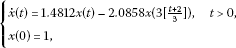(6.1)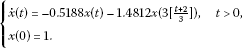(6.2)

In Figure 1-Figure 3, we draw the numerical solutions for Eq. (6.1) and Eq. (6.2), respectively. It is easy to see that the numerical solutions are asymptotically stable.

In order to illustrate the oscillation, we consider the following two problems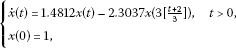(6.3)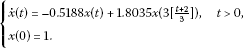(6.4)

In Figure 4-Figure 6, we draw the numerical solutions for Eq. (6.3) and Eq. (6.4), respectively. It is easy to see that the numerical solutions are oscillatory.

## References

1. Busenberg S, Cooke KL: Models of vertically transmitted diseases with sequential-continuous dynamics. In Nonlinear Phenomena in Mathematical Sciences. Edited by: Lakshmikantham V. Academic Press, New York; 1982.

2. Cooke KL, Wiener J: An equation alternately of retarded and advanced type. Proc. Am. Math. Soc. 1987, 99: 726–732. 10.1090/S0002-9939-1987-0877047-8

3. Györi I, Ladas G: Oscillation Theory of Delay Differential Equations. Clarendon Press, Oxford; 1991.

4. Hairer E, Wanner G Stiff and Differential Algebraic Problems. In Solving Ordinary Differential Equations II. Springer, New York; 1993.

5. Liu MZ, Song MH, Yang ZW:Stability of Runge-Kutta methods in the numerical solution of equation ${u}^{\prime }\left(t\right)=au\left(t\right)+{a}_{0}u\left(\left[t\right]\right)$. J. Comput. Appl. Math. 2004, 166: 361–370. 10.1016/j.cam.2003.04.002

6. Liu MZ, Gao JF, Yang ZW: Oscillation analysis of numerical solution in the θ -methods for equation ${x}^{\prime }\left(t\right)+ax\left(t\right)+{a}_{1}x\left(\left[t-1\right]\right)=0$. Appl. Math. Comput. 2007, 186(1):566–578. 10.1016/j.amc.2006.07.119

7. Ran XJ, Liu MZ, Zhu QY: Numerical methods for impulsive differential equation. Math. Comput. Model. 2008, 48: 46–55. 10.1016/j.mcm.2007.09.010

8. Song MH, Yang ZW, Liu MZ: Stability of θ -methods advanced differential equations with piecewise continuous argument. Comput. Math. Appl. 2005, 49: 1295–1301. 10.1016/j.camwa.2005.02.002

9. Wanner G, Hairer E, Nøsett SP: Order stars and stability theorems. BIT Numer. Math. 1978, 18: 475–489. 10.1007/BF01932026

10. Wiener J: Generalized Solutions of Functional Differential Equations. World Scientific, Singapore; 1993.

11. Wiener J, Aftabizadeh AR: Differential equations alternately of retarded and advanced type. J. Math. Anal. Appl. 1988, 129: 243–255. 10.1016/0022-247X(88)90246-6

12. Yang ZW, Liu MZ, Song MH:Stability of Runge-Kutta methods in the numerical solution of equation ${u}^{\prime }\left(t\right)=au\left(t\right)+{a}_{0}u\left(\left[t\right]\right)+{a}_{1}u\left(\left[t-1\right]\right)$. Appl. Math. Comput. 2005, 162: 37–50. 10.1016/j.amc.2003.12.081

## Acknowledgements

The financial support from the National Natural Science Foundation of China (No.11071050) is gratefully acknowledged.

## Author information

Authors

### Corresponding author

Correspondence to Minghui Song.

### Competing interests

The authors declare that they have no competing interests.

### Authors’ contributions

The authors have equal contributions to each part of this article. All the authors read and approved the final manuscript.

## Authors’ original submitted files for images

Below are the links to the authors’ original submitted files for images.

## Rights and permissions

Reprints and Permissions

Song, M., Liu, M. Numerical stability and oscillation of the Runge-Kutta methods for equation ${x}^{\prime }\left(t\right)=ax\left(t\right)+{a}_{0}x\left(M\left[\frac{t+N}{M}\right]\right)$. Adv Differ Equ 2012, 146 (2012). https://doi.org/10.1186/1687-1847-2012-146